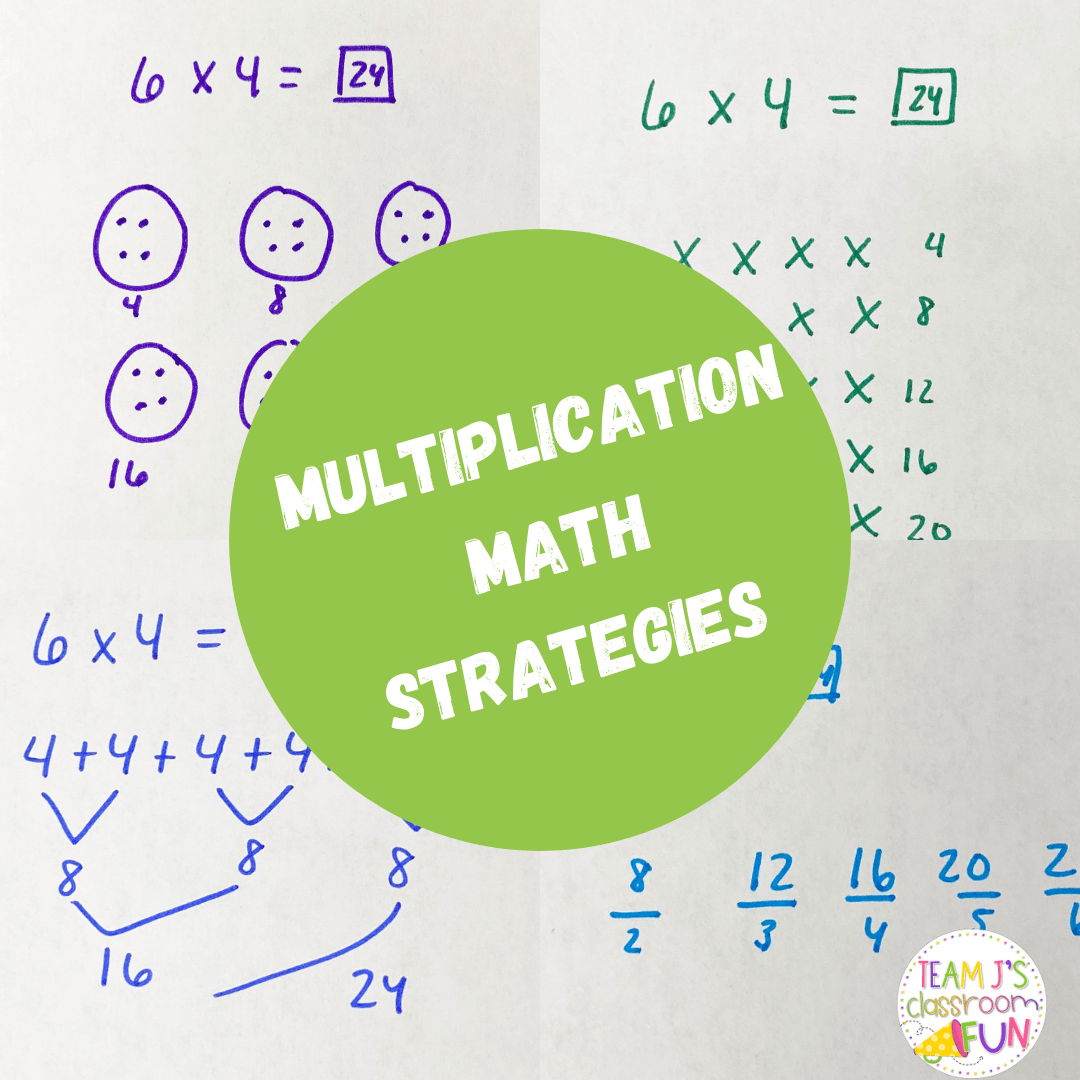Introducing multiplication to my class is one of my favorite math units to teach! I love giving them opportunities to explore the different multiplication math strategies and then finding the one that works best for them!

## Multiplication Math Strategies:

Below you will see four different multiplication math strategies that your students might use.  Each student will be in a different spot with their math strategies. For example, some students need the concrete/manipulatives and equal groups will be best for them.  Other students might be ready for skip counting or repeated addition.  Students can use a variety of strategies and will move through them at different rates when they are ready. I highly recommend letting them explore these strategies first so they understand the concept of multiplication before having them memorize their multiplication facts.

## Equal Groups:

Draw out circles for the number of groups that you have. This problem represents 6 groups of 4. Then, in each group put 4 dots. Then, students will count them. Students can count these in a variety of ways. Some students may count by 1s, 2s, 4s. I recommend having them write the number they are on below each circle to help them keep track. After counting by 2’s, the students came to the answer of 24.

## Arrays:

Arrays are another great visual strategy. Again this problem represents 6 groups of 4. In terms of arrays, this means 6 rows of 4. So students will draw out 6 rows of 4 using “x’s.” Then students count the total to find the answer of 24.

For repeated addition, students will still need to find the answer for 6 groups of 4. So they start by adding 4 six times. Doubles come into play with this, which can make it easier for students to quickly add up the numbers and come to the answer of 24.

## Skip Counting:

For students who are strong with skip counting it can be a great strategy for multiplication. For 6 x4 – students need to skip count 4 six times – 4, 8, 12, 16, 20, 24.

## Multiplication FREEBIE

Grab these FREE Multiplication Word Problem Practice pages for your students! These are great for homework, formative assessments, independent practice, and more! Click HERE to get your FREEBIE!

## Extra Multiplication Practice

If you are interested in more multiplication practice for your students, check out my resources below.

Multiplication Practice Worksheets

Differentiated Multiplication Word Problem Worksheets

Previous Story